# Precalculus : Solving Trigonometric Equations and Inequalities

## Example Questions

2 Next →

### Example Question #1 : Solve Trigonometric Equations And Inequalities In Quadratic Form

Solve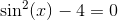when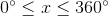Possible Answers:

There are no solutions.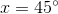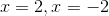Correct answer:

There are no solutions.

Explanation:

Given that, for any input,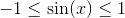, we know that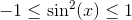, and so the equation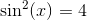can have no solutions.

### Example Question #21 : Trigonometric Identities

Solve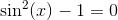whenPossible Answers: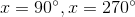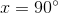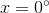There are no solutions.

Correct answer:Explanation:

By adding one to both sides of the original equation, we get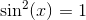, and by taking the square root of both sides of this, we get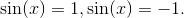From there, we get that, on the given interval, the only solutions areand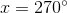.

2 Next →

### All Precalculus Resources